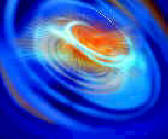#Wordtrade.com Mathematics

### Review Essays of Academic, Professional & Technical Books in the Humanities & Sciences

The Mathematics of Infinity: A Guide to Great Ideas by Theodore G. Faticoni (Pure and Applied Mathematics: A Wiley-Interscience Series of Texts, Monographs and Tracts: Wiley-Interscience) addresses infinite cardinals and is appropriate for readers at any level. Inviting the reader to imagine constructing an infinite chain infinities, which are called cardinals, the author successfully prepares and motivates readers for topics covered within the book. The most unique feature of the book is that set theoretic depth is achieved without losing the target audience. Complementing existing popular books on infinity by actually doing the mathematics involved in addition to talking about the mathematics, the reader is gently led into the world of mathematical proofs.

The most primitive of herdsman used a pouch of stones to keep track of the number of sheep he had in the field. As each sheep would enter the field the herdsman would place a stone in a pile. As the sheep would leave the field the herdsman would place the stones back into the pouch. If there were stones left on the ground then some sheep were missing. I there were no stones left and no sheep left then all was well with the herd. And if there were no more stones but there were more sheep then somehow the herdsman picked up a ewe or two.

This correspondence between pouch stones and sheep is one of the most primitive forms of counting known. In today's language this is known as a one-to-one correspondence, or a bijection between pouch stones and sheep. This kind of counting is continued today when we make an attendance sheet. Each name on the sheet corresponds to exactly one child in the class, and we know some child is missing if he/she does not respond to his/her name. A more important correspondence is found in the grocery store. There we associate a certain number called a price with each item we put in our cart. The items in the cart correspond to a number called the total price of the cart. When we compare our receipt with the objects in the cart we are imitating the sheep herdsman's pouch stones.

The ideal writing style ascribed to by mathematicians is that in writing mathematics, less is more. If we can convey the exact idea of a concept with 5 words instead of 10 then we will use 5 words. Thus we will use the statement Cardinal numbers form a well ordered collection over the wordier statement The well ordered property is enjoyed by the collection of cardinal numbers. The second statement is mathematically correct but it is more than we need to convey the idea.

I have tried to practice this ideal while writing the mathematics in this book. The only exceptions to this ideal are made on the basis of decisions on the educational value of sentence structure, the anecdotal comments, or discussions of this sort that occur between mathematical discourse. Sometimes it is good to sacrifice some mathematical austerity in the interest of getting an important point across to the reader. As the reader will clearly see, this economy of words in mathematical writings is not exercised in the text of a discussion. Discussions and intermediate anecdotes contain examples and illustrations which are the only tools we have to illustrate a concept. Since I have sacrificed a good bit of mathematical rigor in favor of clarity, examples and illustrations are necessary if I am to get some subtle ideas across to the reader. This form of personalized writing style is unavoidable when discussing advanced ideas from mathematics in the popular press.

We have a bit of a mountain to climb in this book, so be patient. Perhaps you can sit down in an overstuffed chair or at a table and open the book. Maybe you have a pencil and paper handy. That's a good idea. Some of these topics need to be diagrammed. And certainly you have a cup of beverage, coffee would be my choice. Now turn on that lamp overhead and blend in the final ingredient: a handful of inspiration. Good luck.

Some foundation has to be laid down before any discussion of mathematics can begin. Our foundation is Set Theory and functions and we will discuss these notions in the first two chapters. There is one assumption that we will use implicitly and explicitly throughout this book. Our underlying assumption is that all mathematical objects considered in this book are from a Mathematical Universe in which these objects exist. Thus when we say that is a cardinal it is to be understood that this cardinal lives in a Mathematical Universe and that we can examine it there. This Mathematical Universe is a classical idea due to the Greek philosopher and mathematician Plato. Thus in making our universal assumption we are following in a good classic tradition. The intent here is clear. We will write given x if we wish to examine an object in the Mathematical Universe. Our definition of Set requires us to know when an object is given. Thus our universal assumption goes to work right away. Whatever else you might believe, let us agree that this Mathematical Universe is there and that we can study the elements in it. Said assumption will not change as we work our way through this book.

Mathematical statements are explicitly or implicitly about elements in sets. One of the highlights of twentieth century mathematics was to show that all of mathematics can be derived from the basic concepts in Set Theory. There is also strong empirical evidence that a firm grounding in Set Theory gives the reader the mathematical experience necessary to understand the advanced abstract ideas covered in later chapters. Thus we begin our introduction to modern mathematics with some work in Set Theory